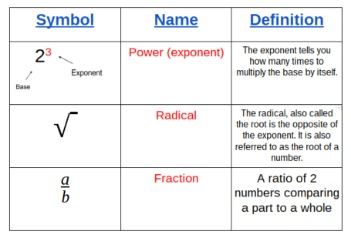# Mathematical Terminology, Concepts & Notation

Instructor: Michelle Vannoy
Have you ever thought that math was too hard to understand, or ever made the statement, ''I just don't get it?'' Maybe the trouble is you don't understand the language. This lesson will break down some of the language of math's most common words and translate it to 'English' for you.

## Math is Like a Foreign Language

Do you speak a foreign language? Suppose someone needed directions but did not speak the same language that you spoke. It would be tough to know what they were asking and even harder to give them the directions they needed to get where they were going. Math is like a foreign language. If you don't speak it, then it's challenging to understand and even harder to follow the directions given. One of the first things you need to do to be a successful math student is to speak and understand the language.

## The Basics

If you have ever learned or tried to learn a new language, you know that you always start with the basics. Let's look at some basic math words and symbols and define what they mean.

• = This is an equal sign. In math, it means that what is on the left of the sign has the same numerical value as what is on the right side of the sign. This could be something simple like 2=2 or something more difficult like y=3x-2. Understanding that no matter which type of statement given, simple or complex, they just mean that the tow statements are the same.
• An equal sign with a slash through it means what is on the left of the equation does not equal what is on the right of the equation. In the case of this sign, the values on each side are different.
• + This is a plus sign, and it means to add. For instance, if you had one apple, and your friend gave you another apple, you know have 2 apples 1+1 = 2. Addition is also referred to as the sum of 2 or more numbers. In the case of addition, you will always end up with more than what you started with. You are gaining more than what you had.
• - This is the symbol for subtraction. Subtraction is used when something is taken away, given away, or spent (in the case of money or bartering). It means to have less than what you started with. It is the opposite operation of addition.
• x or * These are symbols meaning to multiply. Multiplying is adding a number over and over again a specified amount of times. For example, 3*4 would be the same thing as 3+3+3+3. Just like addition, you will be gaining more that what you started with. Multiplication is just a faster way to add.
• / This symbol means to divide. Dividing is placing 'things' into equal groups. For example, 12/4 (12 divided by 4), means to divide 12 into 4 equal groups. If you had 12 objects and divided them into 4 equal groups, you would have 4 groups of 3 items. Division is the opposite operation of multiplication.

These are the basic symbols in math that are used in everyday life.

## Level 1 Unit 2To unlock this lesson you must be a Study.com Member.

### Register to view this lesson

Are you a student or a teacher?

### Unlock Your Education

#### See for yourself why 30 million people use Study.com

##### Become a Study.com member and start learning now.
Back
What teachers are saying about Study.com

### Earning College Credit

Did you know… We have over 160 college courses that prepare you to earn credit by exam that is accepted by over 1,500 colleges and universities. You can test out of the first two years of college and save thousands off your degree. Anyone can earn credit-by-exam regardless of age or education level.